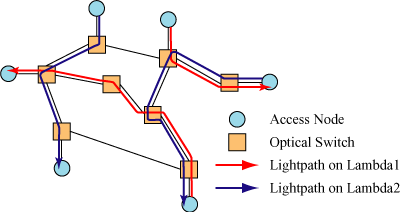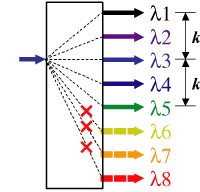# Wavelength Assignment

In all optical WDM networks, wavelength routed networks has benn proposed. In this networks, a lightpath is established between a source node and a destination node whenever a connection request arrives. Lightpath is determined after a selection of physical paths and wavelengths used in data transmission. Without wavelength converters in the networks, wavelength utilization is decreased and the blocking probability is increased for a constraint that the same wavelength must be used in all links between the source node and the destination node(wavelength continuity constraint). In addition, it is not reasonable that all nodes have full wavelength converters because wavelength converters are very expensive. Consequently, many limited wavelength conversion models are researched. Routing and wavelength assignments which decrease the blocking probability has been studied.## Research Introduction

A Wavelength Assignment Scheme for WDM Networks with Limited Range Wavelength Converters
In the wavelength-routed network, the blocking probability can be improved by using wavelength converters because they eliminate the wavelength continuity constraint. Particularly, the network with cost-effective limited-range wavelength converters is a hot topic. In such networks, it is important to consider the range of a wavelength converter in assigning a wavelength. In this paper, we propose a wavelength assignment scheme to consider the number of hops of a connection request to reduce the blocking probability. Simulation results show that the proposed scheme can reduce the wavelength converters by about twenty percent.## Publication

• Sho SHIMIZU, Takanori ITO, Yutaka ARAKAWA, Naoaki YAMANAKA, “A Wavelength Assignment Scheme for WDM Networks with Limited Range Wavelength Converters,” Technical Report IEICE, NS2004-312, pp.329-332, March 2005